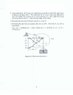# Statics Question - Method of Sections

• wrw103
In summary, Engineering Student is trying to determine the forces in a truss, but is having trouble with the method of sections. He solves the problem by using the method of joints starting from Pulley C, but when he tries to solve the problem using the method of sections, he gets the wrong answer. He is not sure what he is doing wrong, but would appreciate it if someone could help him figure it out. Thanks!f

#### wrw103

Hi everyone,

I was given a statics problem to determine some forces in a truss. The attached image has the specific problem on it. The correct answer was supplied so I know what I should be getting, and I was able to get the correct answer using the method of joints starting from Pulley C. However, when I try to solve the problem using the method of sections, using the cut I have shown on the attached image, I get the wrong answer. I'm not sure what I'm doing wrong, but I would like to understand the correct way to apply the method of sections in this case. The following is what I did to try and determine the forces in two force member AB:

Take moments about point D assuming Tab is in tension with CCW being the positive direction:

Tab*3 + 1500*sin(15)*4+1500*cos(15)*(3+0.5)-1500*(4+0.5) = 0

Solving for Tab:

Tab = -41.99 [lbf] (the negative indicates compression)

The correct answer for Tab is supposed 33.47 [lbf] Tension.

I'm not sure what I'm doing wrong. I would appreciate it if someone could help me figure out where my error so I will have a better understanding of these types of problems.

Thanks,

Engineering Student

#### Attachments

•Statics_Problem_#3.jpg
13.4 KB · Views: 587
By sectioning the structure as shown, you have to take into account more than just AB, you need to look at each truss that is sectioned (including the cable). Can you post the sum equation you're trying to use without any numbers in it?

Hi Mech_Engineer,

I'm using the equation sum of moments = 0, and I'm taking moments about point D. Therefore the tension in member AD and DE have no effect because their lines of action pass through D. So that leaves the tension in AB, the cable pulling downwards, and the cable pulling up at an angle that will create a moment.

$$\Sigma$$MD = 0

Tab*3 + Tcable*sin(15)*4 + Tcable*cos(15)*(3+0.5) - Tcable*(4+0.5) = 0

The tension in the cable will be equal to the weight of box being lifted, 1500 lbf. so the only unknown is AB. I guess one thing I have a question about is where should I consider the force of the cables acting at? I'm assuming the upward cable is acting at the top of the pulley and the downward cable is acting on the right side of the pulley but is that correct?

The terms
Tcable*sin(15)*4 + Tcable*cos(15)*(3+0.5)
are wrong, because you have not taken into account the geometry of the cable wrapped round the pulley. The line of the sloping part does NOT pass through the point at distances 4 and 3.5 from D. It passes through a point that is 6 inches from C at an angle of 15 degrees clockwise from the vertical.

When you solved this by taking moments about C, there is no resultant moment from the cable because both parts of the cable have the same perpendicular distance (6 inches) from C, for any angle of the cable round the pulley, so the math is simpler.

Hi AlephZero,

I think I understand. The cable is acting tangential to the pulley so I cannot use the top of the pulley as a point on the line of action for the tension. I reworked the problem and got the correct answer.

Thanks for the help!